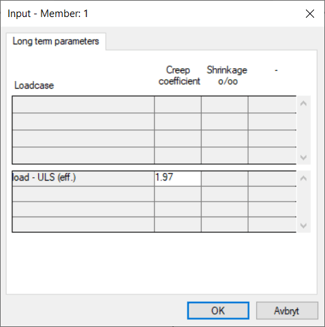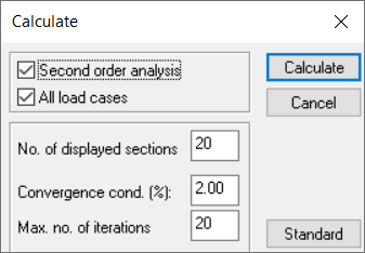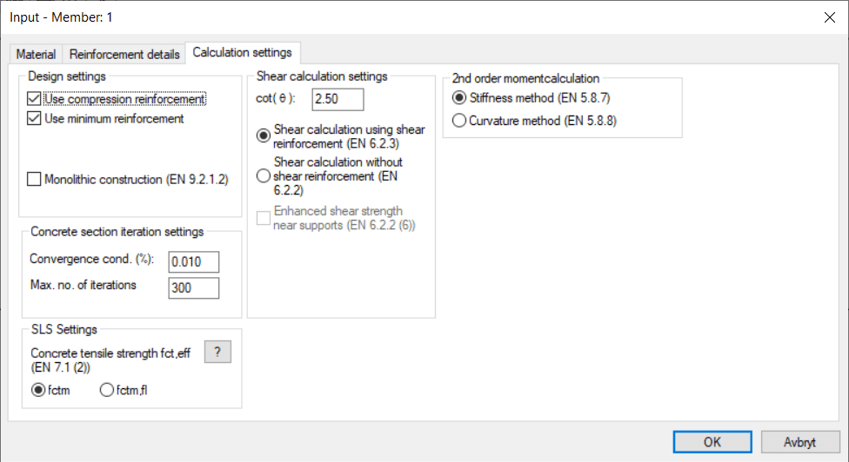# Flexural buckling of concrete members – Three different methods in EuroCode 2

There are three methods to calculate flexural buckling in EuroCode 2. General method according to EN1992-1-1 5.8.6 and the two simplified methods, Stiffness method according to 5.8.7 and Curvature method 5.8.8.

The General method is based on non-linear calculations where a proper 2nd order calculations is performed that at the same time are taking into account the change of stiffness due to cracking of the concrete. The two simplified methods calculate the 2nd order moment using formulas where the parameters has been calibrated using the General method. The General method needs computer calculation ofcourse, whilst the two other can be performed by hand.

When it comes to WIN-Statik, the two programs that handle flexural buckling is Concrete Column and Frame Analysis. Concrete Column can use both the simplified methods while Frame Analysis can manage all three methods.

The three methods can sometimes give different results but are all valid for the Swedish Annex (Check your National Annex - EN1992-1-1 5.8.5 - which methods can be used in your country). It is not possible to say that one method is allways giving the most favourable result, as it may vary with the situation and loading conditions. In all cases it is important to take the effective creep value into account in ULS (Ultimate Limit State).Support cases that we recieve about flexural buckling of concrete usually is about the following two issues:

1. In the first case Frame Analysis has been used to calculate a symetric column, but different results with regards to instability in-plane and out-of-plane, which may be confusing. Usually the user has been using the default setting in the program to design according to the General method in-plane, but according to the Stiffness method out-of-plane. Default value for making a 2nd order analysis is performed since it is activated in the Calculate-window.If a 2nd order analysis is performed, then the General method will be used in-plane, but if it is unchecked the selected simplified method will be used. Out-of-plane the General method can't be used, since this is a plane frame software. In that case one of the simplified methods will be used, where the Stiffness method is default.To get the same result the 2nd order analysis checkbox needs to be inactivated in order for the simplified methods to be used in both directions.

2. The second case is similar, but in this case a calculation has been performed in Concrete Column, where the Stiffness method is default. Then a check in Frame Analysis is made where the 2nd order analysis is active, and a different result is given due to the same reason described in the first case. The solution is to make the 2nd order analysis inactive in Frame to get a corresponding result.

What method that has been used in the calculations are shown in the results.

What method should then be used? There is no general answer to what is best for every occasion as, mentioned above, no method will give the most favourable answer. The General method will be using the most advanced calculations and may be the closest to real life. The reduced stiffness due to cracking often has a large impact on the capacity, which can easily be checked using a similar calculation without cracking in Frame Analysis.

One effect that we have observed during all the years of support around this issue is that when structures with limited amount of reinforcement (i.e. walls), then the Stiffness method gives a significant lower capacity compared to other methods.

J
Joakim is the author of this solution article.

Did you find it helpful? Yes No

Send feedback